# Failure of Clairaut's theorem where only one of the mixed partials is defined

## Statement

### For a function of two variables at a point

It is possible to have a function$f$ of two variables$x,y$ and a point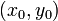$(x_0,y_0)$ in the domain of$f$ such that the second-order mixed partial derivative$f_{xy}(x_0,y_0)$ exists but the second-order mixed partial derivative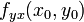$f_{yx}(x_0,y_0)$ does not exist.

## Proof

### Example

Consider a function defined on all of$\R^2$ as: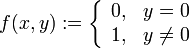$f(x,y) := \left\lbrace \begin{array}{rl} 0, & y = 0 \\ 1, & y \ne 0 \\\end{array} \right.$

We note that:

• Since$f$ depends only on$y$,$f_x(x,y)$ is identically the zero function.
• Thus, the second-order mixed partial derivative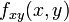$f_{xy}(x,y)$ is identically the zero function.
• On the other hand,$f_y(x,y) = 0$ for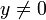$y \ne 0$ and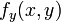$f_y(x,y)$ is undefined for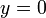$y = 0$.
• In particular, this means that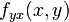$f_{yx}(x,y)$ is not defined on the line$y = 0$ (i.e., the$x$-axis).